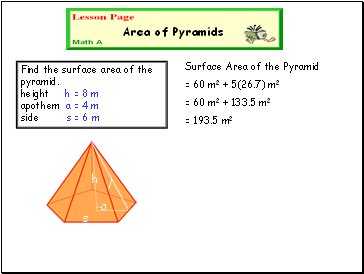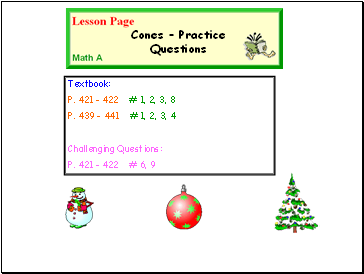# Cones and pyramidsPage 2

#### WATCH ALL SLIDES

l

What shape are the lateral sides?

l 2 = h2 + a2

= 82 + 42

= 80 m2

l = 8.9 m

Attention! the height of the triangle is the slant height ”l ”

= ½ (6)(8.9)

= 26.7 m2

Slide 10Area of Pyramids

Find the surface area of the pyramid. height h = 8 m apothem a = 4 m side s = 6 m

h

a

s

l

Surface Area of the Pyramid

= 60 m2 + 5(26.7) m2

= 60 m2 + 133.5 m2

= 193.5 m2

Slide 11Textbook:

P. 421 - 422 # 1, 2, 3, 8

P. 439 – 441 # 1, 2, 3, 4

Challenging Questions:

P. 421 - 422 # 6, 9

Cones – Practice Questions

Go to page:
1  2